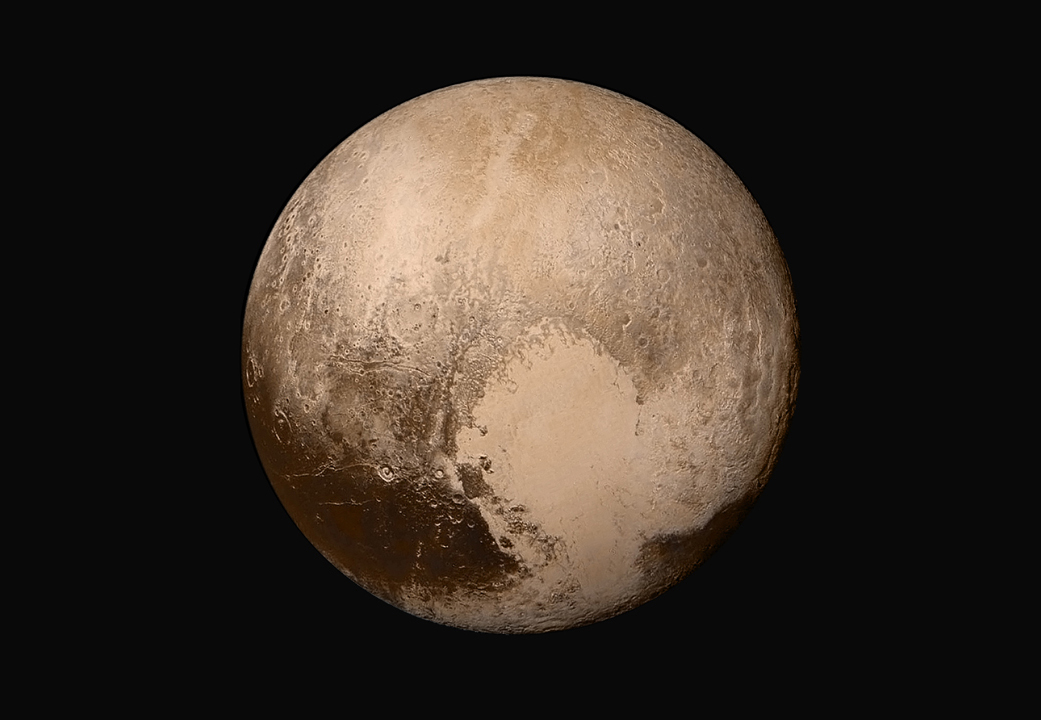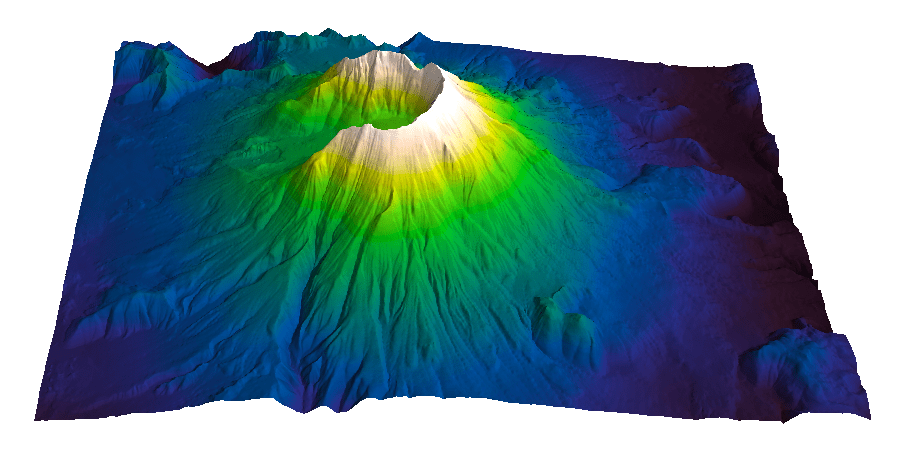# New Horizons truecolor Pluto recolored in Viridis and Inferno

Oh, the new, perceptual MatplotLib colormaps…..

Here’s one stunning, recent Truecolor image of Pluto from the New Horizons mission:Original image: The Rich Color Variations of Pluto. Credit: NASA/JHUAPL/SwRI. Click on the image to view the full feature on New Horizon’s site

Below, I recolored using two of the new colormaps:Recolored images: I like Viridis, by it is Inferno that really brings to life this image, because of its wider hue and lightness range!

# Visualize Mt St Helen with Python and a custom color palette

Evan Bianco of Agile Geoscience wrote a wonderful post on how to use python to import, manipulate, and display digital elevation data for Mt St Helens before and after the infamous 1980 eruption. He also calculated the difference between the two surfaces to calculate the volume that was lost because of the eruption to further showcase Python’s capabilities. I encourage readers to go through the extended version of the exercise by downloading his iPython Notebook and the two data files here and here.

I particularly like Evan’s final visualization (consisting of stacked before eruption, difference, and after eruption surfaces) which he created in Mayavi, a 3D data visualization module for Python. So much so that I am going to piggy back on his work, and show how to import a custom palette in Mayavi, and use it to color one of the surfaces.

#### Python Code

This first code block imports the linear Lightness palette. Please refer to my last post for instructions on where to download the file from.

```import numpy as np
# load 256 RGB triplets in 0-1 range:
# create r, g, and b 1D arrays:
r=LinL[:,0]
g=LinL[:,1]
b=LinL[:,2]
# create R,G,B, and ALPHA 256*4 array in 0-255 range:
r255=np.array([floor(255*x) for x in r],dtype=np.int)
g255=np.array([floor(255*x) for x in g],dtype=np.int)
b255=np.array([floor(255*x) for x in b],dtype=np.int)
a255=np.ones((256), dtype=np.int); a255 *= 255;
RGBA255=zip(r255,g255,b255,a255)```

This code block imports the palette into Mayavi and uses it to color the Mt St Helens after the eruption surface. You will need to have run part of Evan’s code to get the data.

```from mayavi import mlab
# create a figure with white background
mlab.figure(bgcolor=(1, 1, 1))
# create surface and passes it to variable surf
surf=mlab.surf(after, warp_scale=0.2)
# import palette
surf.module_manager.scalar_lut_manager.lut.table = RGBA255
# push updates to the figure
mlab.draw()
mlab.show()
```#### Reference

Mayavi custom colormap example

#### Related Posts (MyCarta)

The rainbow is dead…long live the rainbow! – Part 5 – CIE Lab linear L* rainbow

Convert color palettes to Python Matplotlib colormaps

#### Related Posts (external)

How much rock was erupted from Mt St Helens

# Convert color palettes to python matplotlib colormaps

This is a quick post to show you how to import my perceptual color palettes – or any other color palette – into Python and convert them into Matplotlib colormaps. We will use as an example the CIE Lab linear L* palette, which was my adaptation to Matlab of the luminance controlled colormap by Kindlmann et al.

#### Introduction

You can use the code snippets in here or download the iPython notebook from here (*** please see update ***). You will need NumPy in addition to Matplotlib.

#### ***  UPDATE  ***

Fellow Pythonista Matt Hall of Agile Geoscience extended this work – see comment below to include more flexible import of the data and formatting routines, and code to reverse the colormap. Please use Matt’s expanded iPython notebook.

#### Preliminaries

First of all, get the color palettes in plain ASCII format rom this page. Download the .odt file for the RGB range 0-1 colors, change the file extension to .zip, and unzip it. Next, open the file Linear_L_0-1, which contains comma separated values, replace commas with tabs, and save as .txt file. That’s it, the rest is in Python.

#### Code snippets – importing data

Let’s bring in numpy and matplotlib:

```%pylab inline
import numpy as np
%matplotlib inline
import matplotlib.pyplot as plt```

Now we load the data. We get RGB triplets from tabs delimited text file. Values are expected in the 0-1 range, not 0-255 range.

`LinL = np.loadtxt('Linear_L_0-1.txt')`

A quick QC of the data:

`LinL[:3]`

gives us:

```array
([[ 0.0143,  0.0143,  0.0143],
[ 0.0404,  0.0125,  0.0325],
[ 0.0516,  0.0154,  0.0443]])```

looking good!

#### Code snippets – creating colormap

Now we want to go from an array of values for blue in this format:

`b1=[0.0000,0.1670,0.3330,0.5000,0.6670,0.8330,1.0000]`

to a list that has this format:

```[(0.0000,1.0000,1.0000),
(0.1670,1.0000,1.0000),
(0.3330,1.0000,1.0000),
(0.5000,0.0000,0.0000),
(0.6670,0.0000,0.0000),
(0.8330,0.0000,0.0000),
(1.0000,0.0000,0.0000)]```

# to a dictionary entry that has this format:

```{
'blue': [
(0.0000,1.0000,1.0000),
(0.1670,1.0000,1.0000),
(0.3330,1.0000,1.0000),
(0.5000,0.0000,0.0000),
(0.6670,0.0000,0.0000),
(0.8330,0.0000,0.0000),
(1.0000,0.0000,0.0000)],
...
...
}```

which is the format required to make the colormap using matplotlib.colors. Here’s the code:

```b3=LinL[:,2] # value of blue at sample n
b2=LinL[:,2] # value of blue at sample n
b1=linspace(0,1,len(b2)) # position of sample n - ranges from 0 to 1

# setting up columns for list
g3=LinL[:,1]
g2=LinL[:,1]
g1=linspace(0,1,len(g2))

r3=LinL[:,0]
r2=LinL[:,0]
r1=linspace(0,1,len(r2))

# creating list
R=zip(r1,r2,r3)
G=zip(g1,g2,g3)
B=zip(b1,b2,b3)

# transposing list
RGB=zip(R,G,B)
rgb=zip(*RGB)
# print rgb

# creating dictionary
k=['red', 'green', 'blue']
LinearL=dict(zip(k,rgb)) # makes a dictionary from 2 lists

```

#### Code snippets – testing colormap

That’s it, with the next 3 lines we are done:

```my_cmap = matplotlib.colors.LinearSegmentedColormap('my_colormap',LinearL)
pcolor(rand(10,10),cmap=my_cmap)
colorbar()```

which gives you this result:Notice that if the original file hadn’t had 256 samples, and you wanted a 256 sample color palette, you’d use the line below instead:

`my_cmap = matplotlib.colors.LinearSegmentedColormap('my_colormap',LinearL,256)`

Have fun!

#### Related Posts (MyCarta)

The rainbow is dead…long live the rainbow! – Part 5 – CIE Lab linear L* rainbow

Visualize Mt S Helens with Python and a custom color palette

#### A bunch of links on colors in Python

http://bl.ocks.org/mbostock/5577023

http://matplotlib.org/api/cm_api.html

http://matplotlib.org/api/colors_api.html

http://matplotlib.org/examples/color/colormaps_reference.html

http://matplotlib.org/api/colors_api.html#matplotlib.colors.ListedColormap

http://wiki.scipy.org/Cookbook/Matplotlib/Show_colormaps

http://stackoverflow.com/questions/21094288/convert-list-of-rgb-codes-to-matplotlib-colormap

http://stackoverflow.com/questions/16834861/create-own-colormap-using-matplotlib-and-plot-color-scale

http://stackoverflow.com/questions/11647261/create-a-colormap-with-white-centered-around-zero